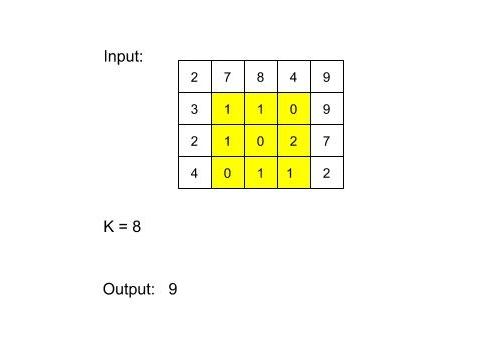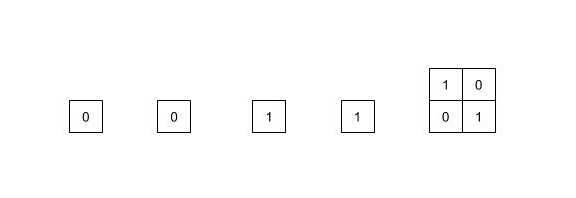0

# Square Submatrix with sum less than or equal to K

Difficulty: MEDIUM
Avg. time to solve
30 min
Success Rate
70%

Problem Statement
Suggest Edit

#### For example :#### If there is no square sub-matrix with sum less than or equal to K, then return 0.

##### Input Format
``````The first line of input contains an integer 'T' representing the number of test cases. Then the test cases follow.

The first line of each test case contains three single-spaced integers N, M and K, representing the number of rows, number of columns and the given integer, respectively.

The next N line contains M single-spaced elements.
``````
##### Output Format:
``````For each test case, print the size of the largest sub-matrix with sum less than or equal to K.

The output for each test case is in a separate line.
``````
##### Note:
``````You do not need to print anything, it has already been taken care of. Just implement the given function.
``````
##### Constraints:
``````1 <= T <= 5
1 <= N, M <= 100
0 <= K <= 10^5,
0 <= data <= 10^5,

where ‘T’ is the number of test cases,  ‘N’ and ‘M’ are the numbers of rows and columns respectively and ‘data’ is the value of the elements of the matrix.
``````
##### Sample Input 1:
``````3
3 3 2
1 0 3
0 1 5
4 4 5
2 2 9
1 2
0 5
1 2 1
5 3
``````
##### Sample Output 1:
``````4
4
0
``````
##### Explanation of Sample Input 1:
``````In the 1st test case, there are four square submatrices of size 1(1 x 1) and one square sub-matrix of size 4(2 x 2) with sum less than or equal to K.
````````````In the 2nd test case, the whole matrix has sum 8 which is less than 9.
In the 3rd test case, there is no square sub-matrix with sum less than or equal to K.
``````
##### Sample Input 2:
``````2
3 3 3
4 4 4
2 1 1
0 0 1
1 1 1
0
``````
##### Sample Output 2:
``````4
1
``````Want to solve this problem? Login now to get access to solve the problems